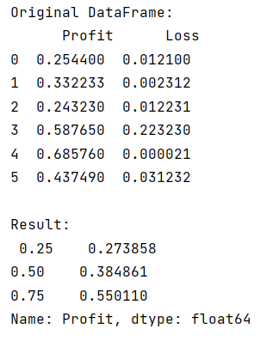# Python Pandas: How to calculate 1st and 3rd quartiles?

Given a Pandas DataFrame, we have to calculate 1st and 3rd quartiles.
Submitted by Pranit Sharma, on July 28, 2022

Pandas is a special tool that allows us to perform complex manipulations of data effectively and efficiently. Inside pandas, we mostly deal with a dataset in the form of DataFrame. DataFrames are 2-dimensional data structures in pandas. DataFrames consist of rows, columns, and data.

Pandas consist of almost every kind of logical and mathematical operation. It allows us to calculate different statistical expressions from the DataFrame.

Quantiles are the set of values that is divided into equal-sized and equal-frequency subgroups.

Here, we will learn to calculate 1st and 3rd quantiles in a DataFrame. The quantiles are usually divided into a sub-group of 25%, 50%, and 75%.

Pandas have a method called quantile() which takes a list of all the quantiles we want as an argument. We pass the quantiles in decimal form, for instance, 25% will be passed as 0.25

Let us understand with the help of an example,

## Python code to calculate 1st and 3rd quartiles

```# Importing pandas package
import pandas as pd

# Creating a Dictionary
data = {
'Profit':[0.2544,0.332233,0.24323,0.58765,0.68576,0.43749],
'Loss':[0.0121,0.0023123,0.012231,0.22323,0.000021,0.0312321]
}

# Creating a DataFrame
df = pd.DataFrame(data)

# Display DataFrame
print("Original DataFrame:\n",df,"\n")

# Calculating quantiles
result = df.Profit.quantile([0.25,0.5,0.75])

# Display result
print("Result:\n",result)
```

Output:Languages: » C » C++ » C++ STL » Java » Data Structure » C#.Net » Android » Kotlin » SQL
Web Technologies: » PHP » Python » JavaScript » CSS » Ajax » Node.js » Web programming/HTML
Solved programs: » C » C++ » DS » Java » C#
Aptitude que. & ans.: » C » C++ » Java » DBMS
Interview que. & ans.: » C » Embedded C » Java » SEO » HR
CS Subjects: » CS Basics » O.S. » Networks » DBMS » Embedded Systems » Cloud Computing
» Machine learning » CS Organizations » Linux » DOS
More: » Articles » Puzzles » News/Updates